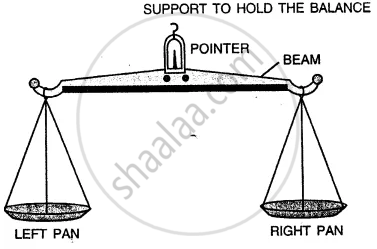Share

# Name the Instrument Which is Commonly Used to Measure the Mass of a Body. State How is It Used ? - Physics

Course
ConceptMeasurement of Mass (Beam Balance, Electronic Balance).

#### Question

Name the instrument which is commonly used to measure the mass of a body. State how is it used ?

#### Solution

Instrument commonly used to measure the mass of a body, is the beam balance.When we hold up the balance, we observe that when there is nothing on either pan, the beam is horizontal. The body whose mass is to be measured is placed on the left pan. The standard weight are put on the right pan. They are so adjusted that the beam is again horizontal on holding the balance up. The total of the standard weights gives the mass of the given body.

Is there an error in this question or solution?

#### APPEARS IN

Solution Name the Instrument Which is Commonly Used to Measure the Mass of a Body. State How is It Used ? Concept: Measurement of Mass (Beam Balance, Electronic Balance)..
S